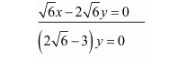# Solve the following systems of equations:

Question:

Solve the following systems of equations:

$\sqrt{2} x-\sqrt{3} y=0$

$\sqrt{3} x-\sqrt{8} y=0$

Solution:

The given equations are:

$\sqrt{2} x-\sqrt{3} y=0 \ldots(i)$

$\sqrt{3} x-\sqrt{8} y=0 \ldots($ ii $)$

Multiply equation $(i)$ by $\sqrt{3}$ and equation $(i i)$ by $\sqrt{2}$ and subtract equation (ii) from (i), we get

$\sqrt{6} x-3 y=0$$\Rightarrow y=0$

Put the value of $y$ in equation $(i)$, we get

$\sqrt{2} x+0 \times y=0$

$\Rightarrow x=0$

Hence the value of $x=0$ and $y=0$